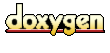Main Page   Modules   Class Hierarchy   Alphabetical List   Compound List   File List   Compound Members   File Members

Fl_Date_Time Class Reference

Fl_Date_Time. More...

`#include <Fl_Date_Time.h>`

List of all members.

Public Methods

Fl_Date_Time (short y, short m, short d, short hour=0, short minute=0, short second=0)
Fl_Date_Time (const char *dat)
Fl_Date_Time (const Fl_Date_Time &dt)
Fl_Date_Time (const double dt=0)
void format_date (char *str) const
void format_time (char *str, bool ampm=true) const
short days_in_month () const
short day_of_week () const
short day_of_year () const
Fl_String day_name () const
Fl_String month_name () const
unsigned date () const
short day () const
short month () const
short year () const
Fl_String date_string () const
Fl_String time_string () const
void decode_date (short *y, short *m, short *d) const
void decode_time (short *h, short *m, short *s, short *ms) const
operator double (void) const
void operator= (const Fl_Date_Time &date)
void operator= (const char *dat)
Fl_Date_Time operator+ (int i)
Fl_Date_Time operator- (int i)
Fl_Date_Time operator+ (Fl_Date_Time &dt)
Fl_Date_Time operator- (Fl_Date_Time &dt)
Fl_Date_Time & operator+= (int i)
Fl_Date_Time & operator-= (int i)
Fl_Date_Time & operator+= (Fl_Date_Time &dt)
Fl_Date_Time & operator-= (Fl_Date_Time &dt)
Fl_Date_Time & operator++ ()
Fl_Date_Time & operator++ (int)
Fl_Date_Time & operator-- ()
Fl_Date_Time & operator-- (int)

Static Public Methods

Fl_Date_Time convert (const long)
void Now (Fl_Date_Time dt)
Fl_Date_Time System ()
Fl_Date_Time Now ()
Fl_Date_Time Date ()
Fl_Date_Time Time ()
void decode_date (const double dt, short &y, short &m, short &d)
void decode_time (const double dt, short &h, short &m, short &s, short &ms)
void encode_date (double &dt, short y=0, short m=0, short d=0)
void encode_date (double &dt, const char *dat)
void encode_time (double &dt, short h=0, short m=0, short s=0, short ms=0)
void encode_time (double &dt, const char *tim)
bool is_leap_year (const short year)

Static Public Attributes

char dateInputFormat 
char timeInputFormat 
char dateFormat 
char timeFormat 
char datePartsOrder 
char dateSeparator
char timeSeparator
bool time24Mode

Protected Attributes

double m_dateTime

Static Protected Attributes

double dateTimeOffset

Detailed Description

Fl_Date_Time.

Definition at line 29 of file Fl_Date_Time.h.

The documentation for this class was generated from the following file:
Generated on Thu Jul 31 15:33:47 2003 for eFLTK by1.2.15